load("workspace.RData")
library(fpp2)
## Warning: package 'fpp2' was built under R version 3.6.1
## Loading required package: ggplot2
## Registered S3 methods overwritten by 'ggplot2':
##   method         from
##   [.quosures     rlang
##   c.quosures     rlang
##   print.quosures rlang
## Loading required package: forecast
## Warning: package 'forecast' was built under R version 3.6.1
## Registered S3 method overwritten by 'xts':
##   method     from
##   as.zoo.xts zoo
## Registered S3 method overwritten by 'quantmod':
##   method            from
##   as.zoo.data.frame zoo
## Registered S3 methods overwritten by 'forecast':
##   method             from
##   fitted.fracdiff    fracdiff
##   residuals.fracdiff fracdiff
## Loading required package: fma
## Warning: package 'fma' was built under R version 3.6.1
## Loading required package: expsmooth
## Warning: package 'expsmooth' was built under R version 3.6.1

Simple Forecasting Methods

1. Average Method

It is the average of the historical data. meanf(y, h) y contains the time series h is the forecast horizon

1. Naive method

We simply set all forecasts to the value of last observation. Works remarkably well for many economic and financial time series. It is optimal when data follows a random walk, these are also called random walk forecasts.

naive(y, h) rwf(y, h) # Equivalent alternative

1. Seasonal Naive Method

Useful for highly seasonal data. We set each forecast to be equal to the last observed value from the same season of the year.

snaive(y, h)

1. Drift Method

Allow the forecast to increase of decrease over time, where the amount of change over time ( called the drift) is set to be the average change seen in the historical data.

rwf(y, h, drift=TRUE)

Example: Quarterly Beer Production

# Set training data from 1992 to 2007
beer2 <- window(ausbeer,start=1992,end=c(2007,4))
# Plot some forecasts
autoplot(beer2) +
autolayer(meanf(beer2, h=11),
series="Mean", PI=FALSE) +
autolayer(naive(beer2, h=11),
series="Naïve", PI=FALSE) +
autolayer(snaive(beer2, h=11),
series="Seasonal naïve", PI=FALSE) +
ggtitle("Forecasts for quarterly beer production") +
xlab("Year") + ylab("Megalitres") +
guides(colour=guide_legend(title="Forecast"))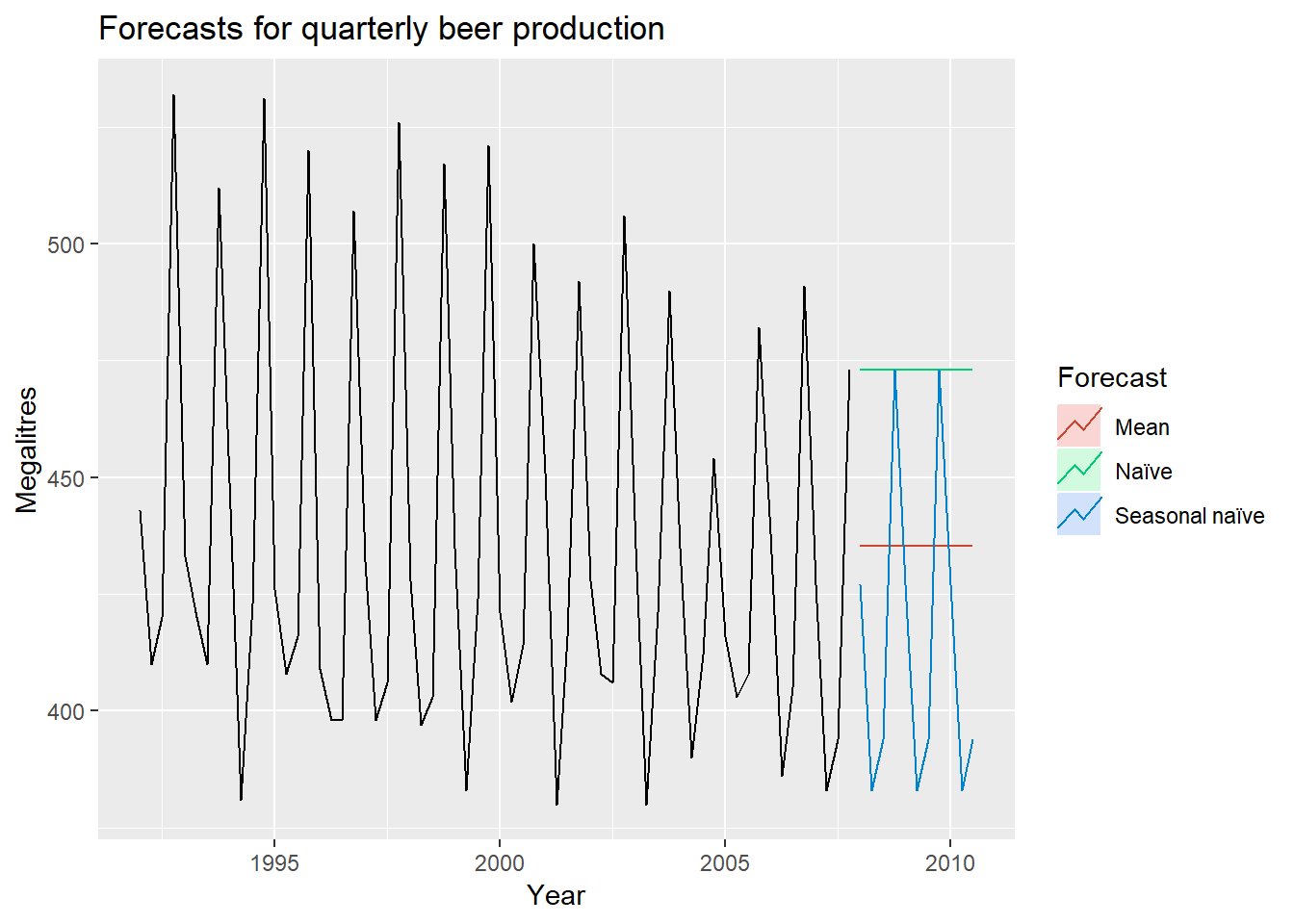these methods will serve as benchmarks rather than the method of choice. That is, any forecasting methods we develop will be compared to these simple methods to ensure that the new method is better than these simple alternatives. If not, the new method is not worth considering.

## Mathematical Transformation

If the data show variation that increases or decreases with the level of the series, then a transformation can be useful. For example, a logarithmic transformation is often useful.Logarithms are useful because they are interpretable: changes in a log value are relative (or percentage) changes on the original scale.Another useful feature of log transformations is that they constrain the forecasts to stay positive on the original scale.

A useful family of transformations, that includes both logarithms and power transformations, is the family of Box-Cox transformations, which depend on the parameter lambda. when lambda=0, natural log are used, when lambda is not equal to zero power transformations are used. When lamdba is 1, the transformed data is shifted downwards, but there is no change in shape. You can use BoxCox.lambda()to choose the value autometically.

(lambda <- BoxCox.lambda(elec))
##  0.2654076

Now you can plot using this value

autoplot(BoxCox(elec,lambda))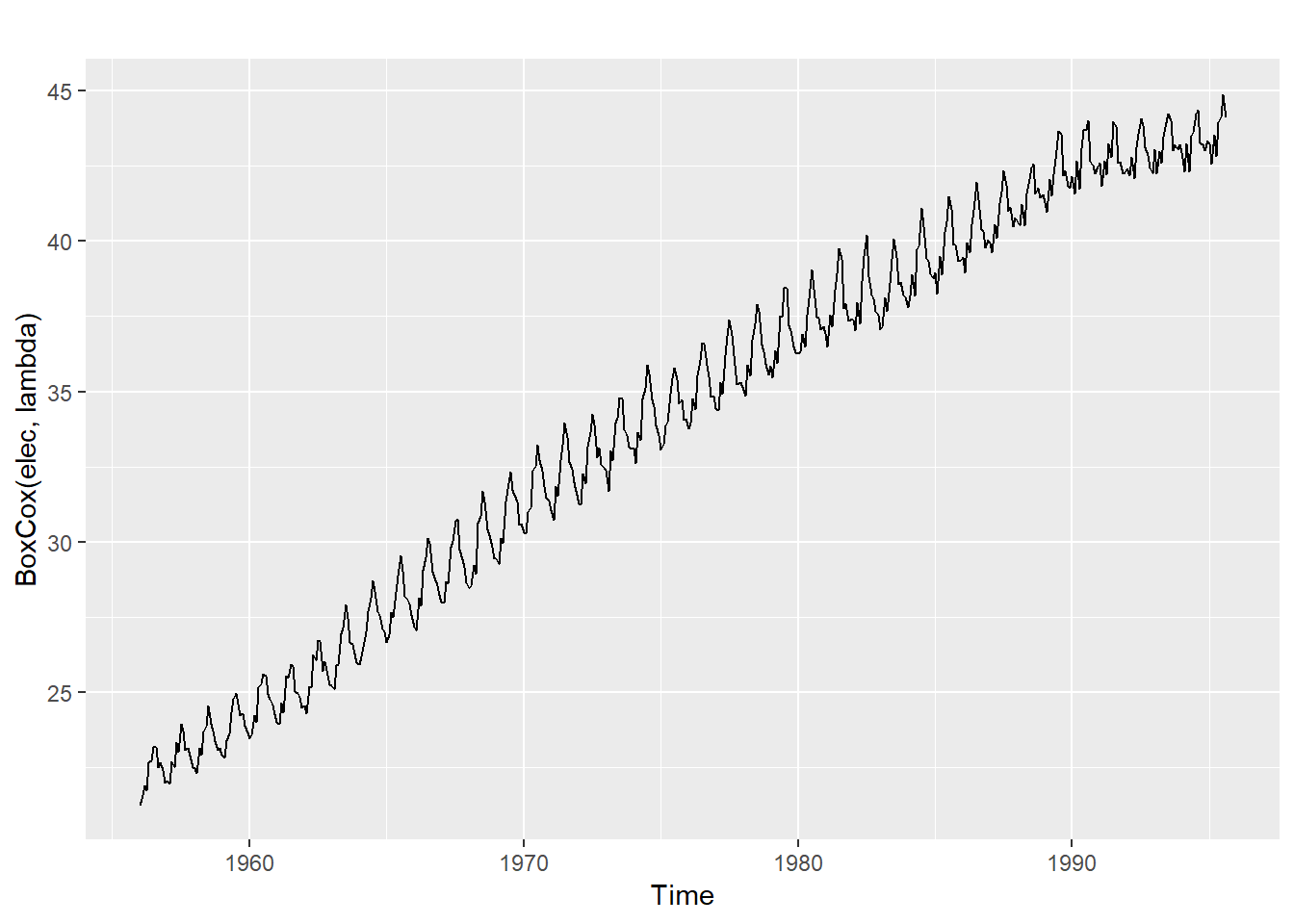One issue with using mathematical transformations such as Box-Cox transformations is that the back-transformed forecast will not be the mean of the forecast distribution. When doing forecasting with this, we need to back transform it, but there will be bias. For that we need bias adjustment

The example below gives both the results: simple back transformation and bias adjusted transformation Data is average annual price of eggs using the drift method with a log transformation ( lambad=o)

fc <- rwf(eggs, drift=TRUE, lambda=0, h=50, level=80)
fc2 <- rwf(eggs, drift=TRUE, lambda=0, h=50, level=80,
autoplot(eggs) +
autolayer(fc, series="Simple back transformation") +
guides(colour=guide_legend(title="Forecast"))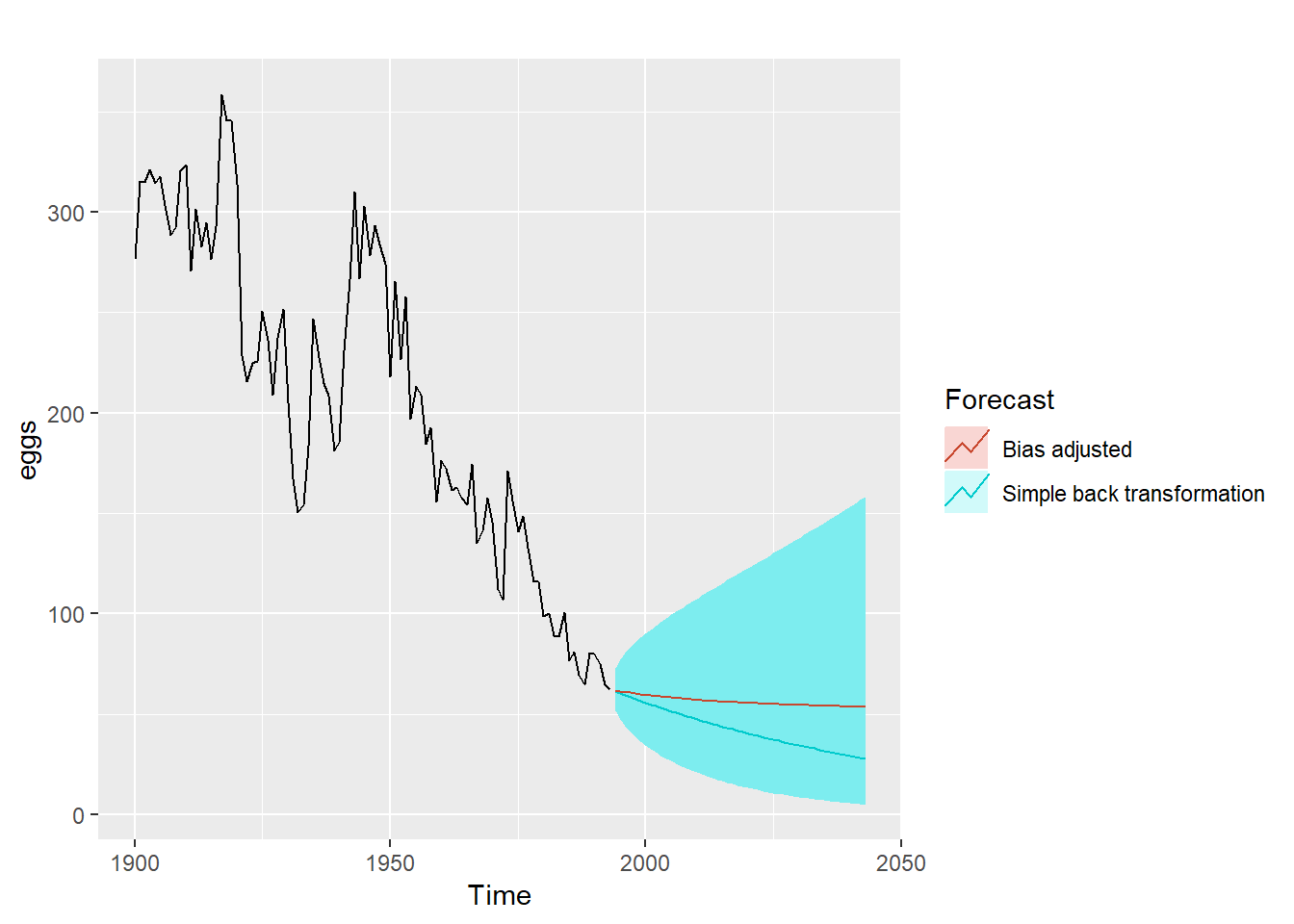## Residual Test

The residuals should 1. be uncorrelated 2. Have zero mean 3. Constrant Variance 4. Normally Distributed

autoplot(goog200) +
xlab("Day") + ylab("Closing Price (US$)") + ggtitle("Google Stock (daily ending 6 December 2013)")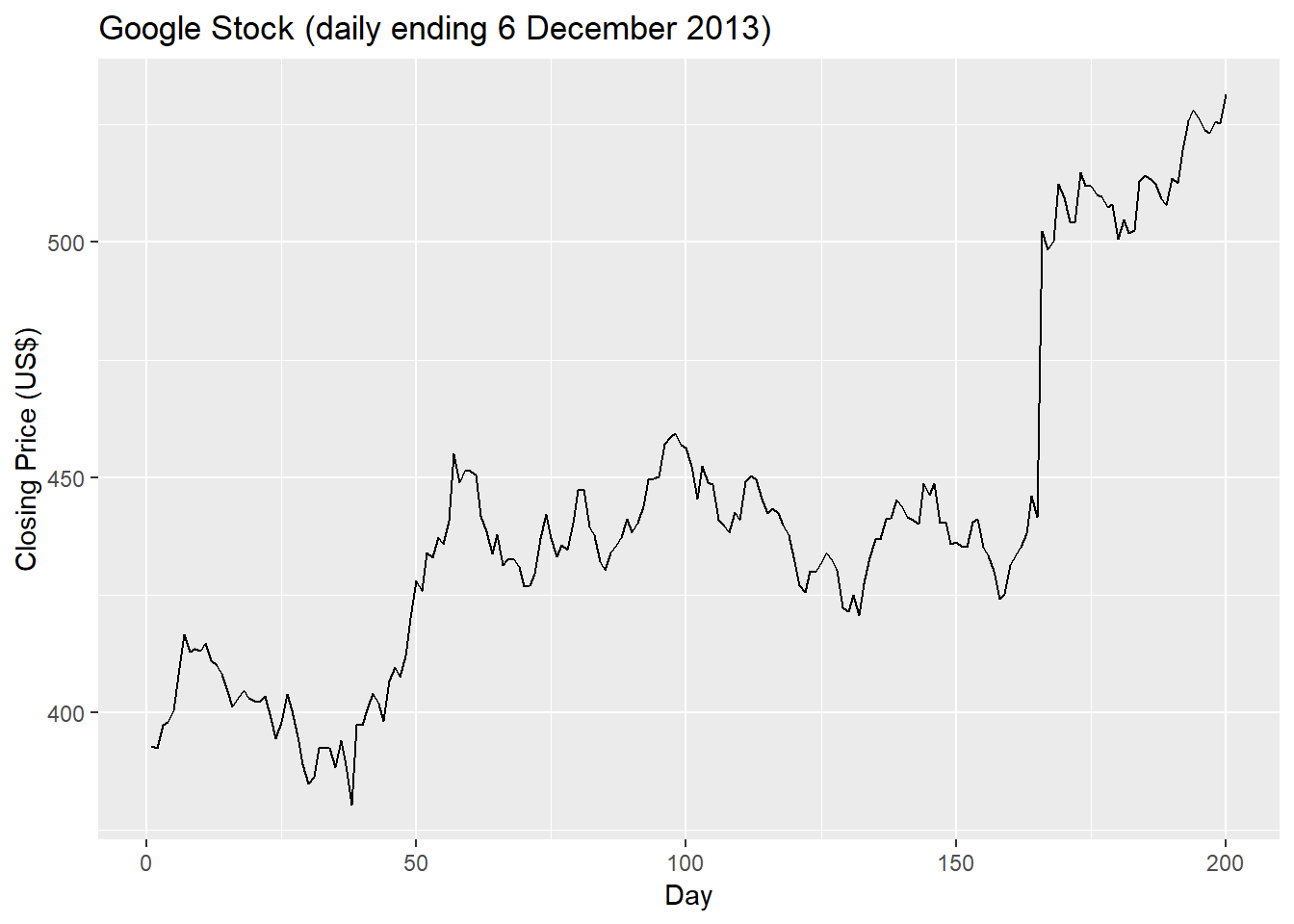Lets use the Naive method of forecasting and get the residuals res <- residuals(naive(goog200)) autoplot(res) + xlab("Day") + ylab("") + ggtitle("Residuals from naïve method")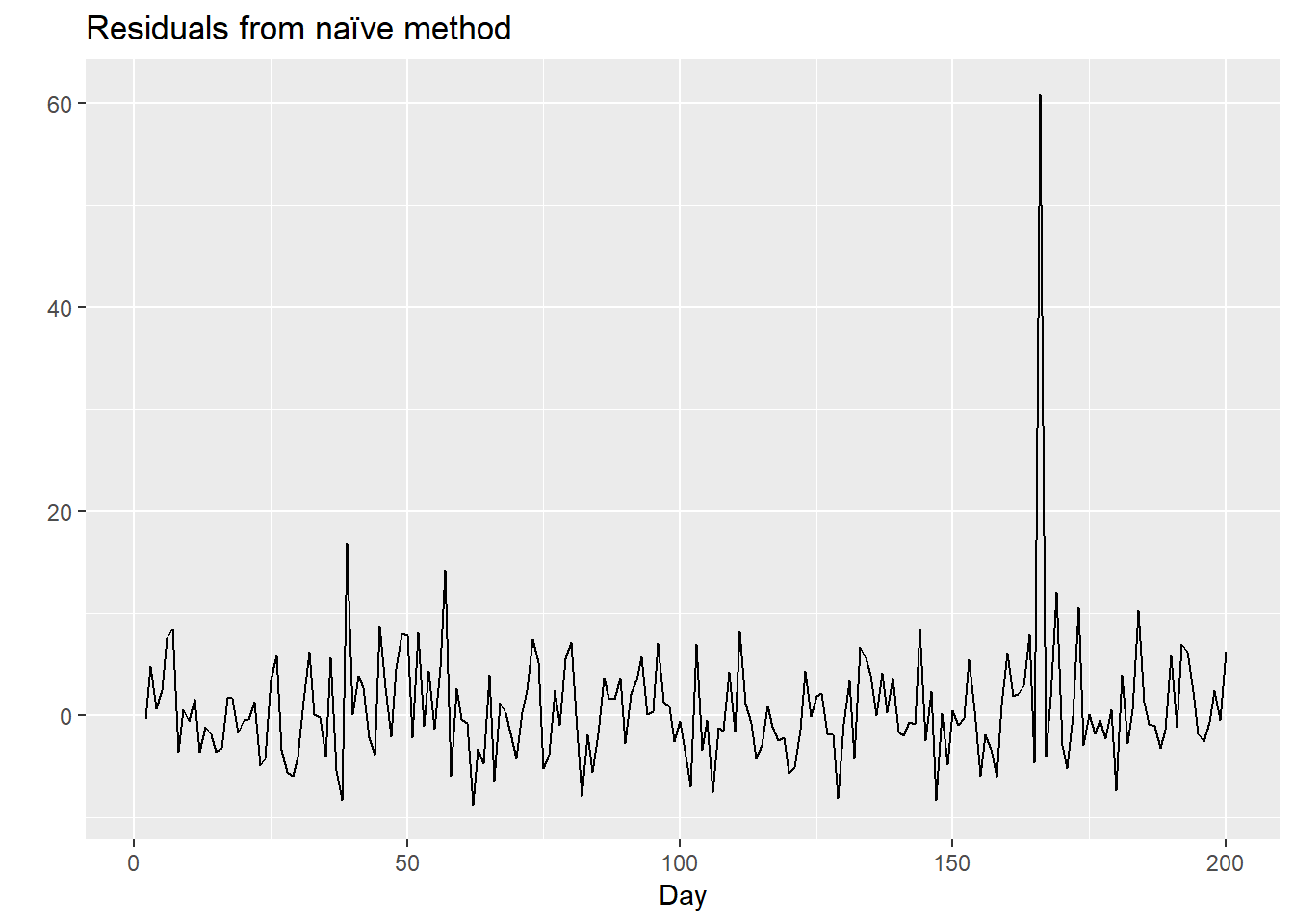The time plot suggests that the variation of the residuals stays much the same across historical data, apart from one outlier. Lets Check Histogram gghistogram(res) + ggtitle("Histogram of residuals") ## Warning: Removed 1 rows containing non-finite values (stat_bin).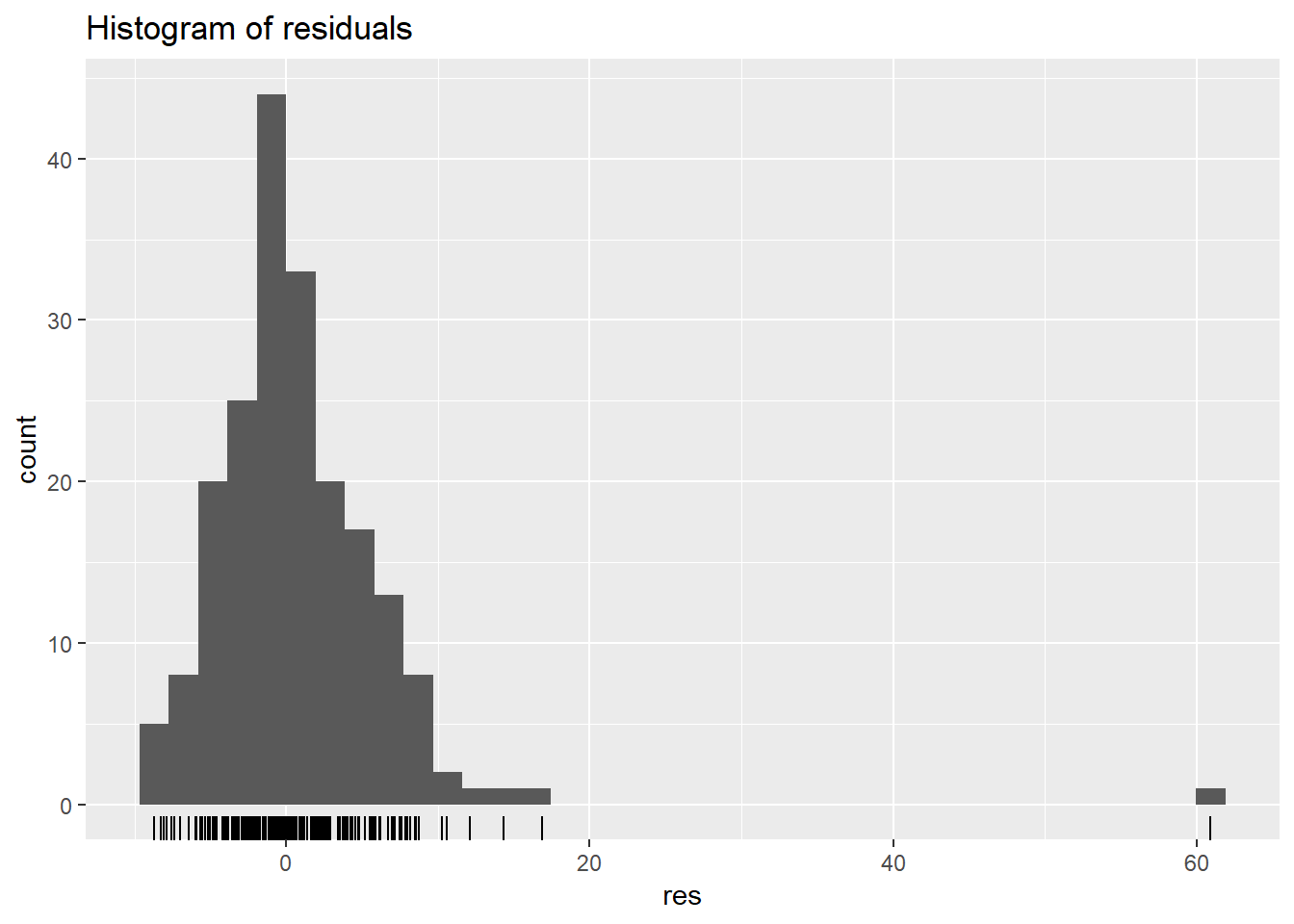The right Tail seems too long for normal distribution. the mean is close to zero. lets get the ACF of residuals ggAcf(res) + ggtitle("ACF of residuals")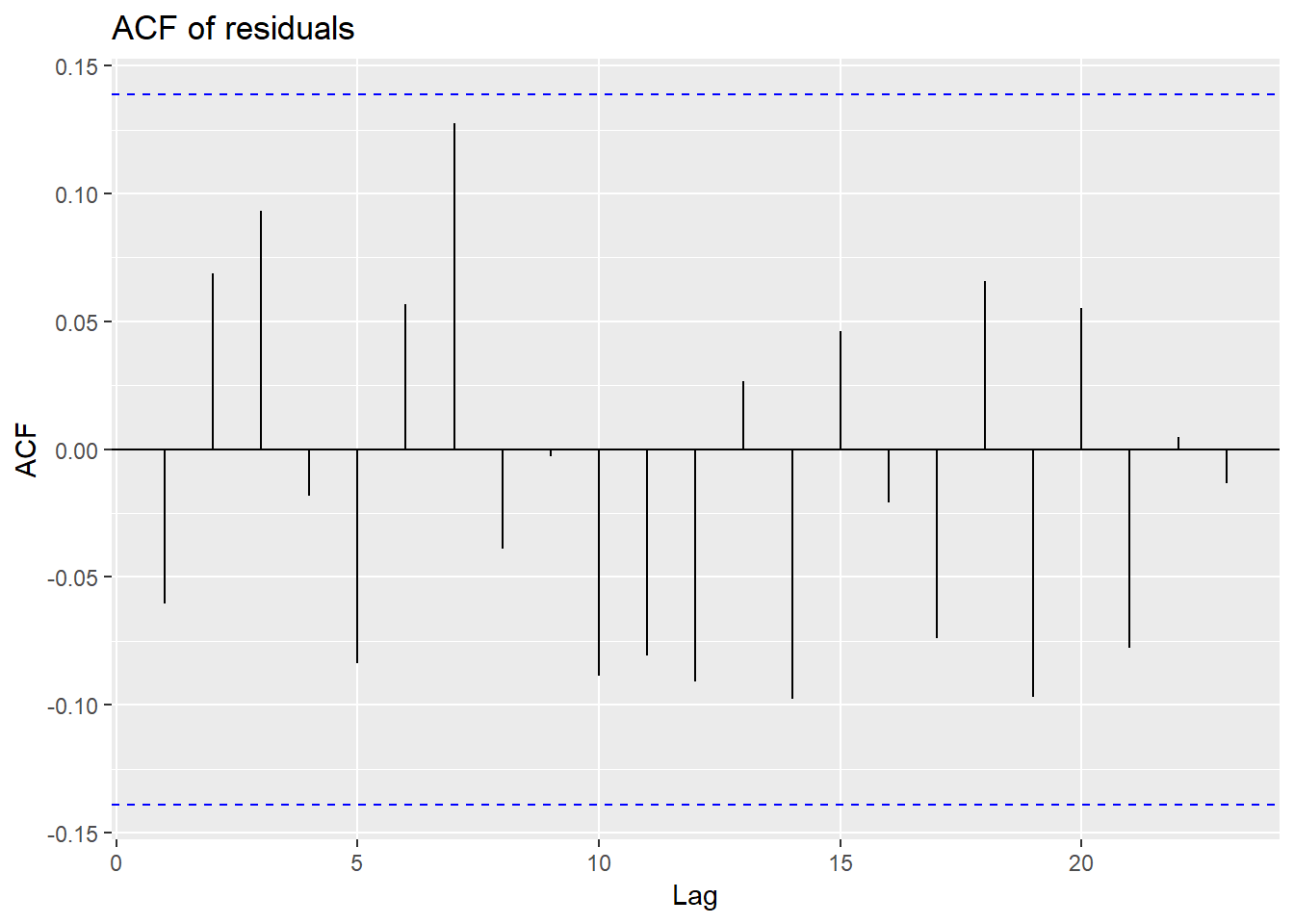The lack of correlation suggests that the forecast is good. Overall prediction intervals that are computed assuming normal distribution may be inaccurate. We need also to see whether the first h autocorrelations are significantly different from the white noise. Two tests Box Pierce and Ljung Box tests are used . h(lag) should be 10 for non seasonal data and 2m for seasonal where m is the period of seasonality, but should not be more than T/5 where T is the number of observations. K is the number of parameters in the model. Set the value of K(fitdf) =0 ( if it is coming from raw data). Using the tests on the residuals we have Box.test(res, lag=10, fitdf=0) ## ## Box-Pierce test ## ## data: res ## X-squared = 10.611, df = 10, p-value = 0.3886 #Box pierce test Large value of p indicate no correlation and residuals are not distinguishable from white noise. Box.test(res,lag=10, fitdf=0, type="Lj") ## ## Box-Ljung test ## ## data: res ## X-squared = 11.031, df = 10, p-value = 0.3551 Again large value of p indicate no correlation and residuals are not distinguishable from white noise. ### you can check all of these with checkresiduals()funciton of R checkresiduals(naive(goog200))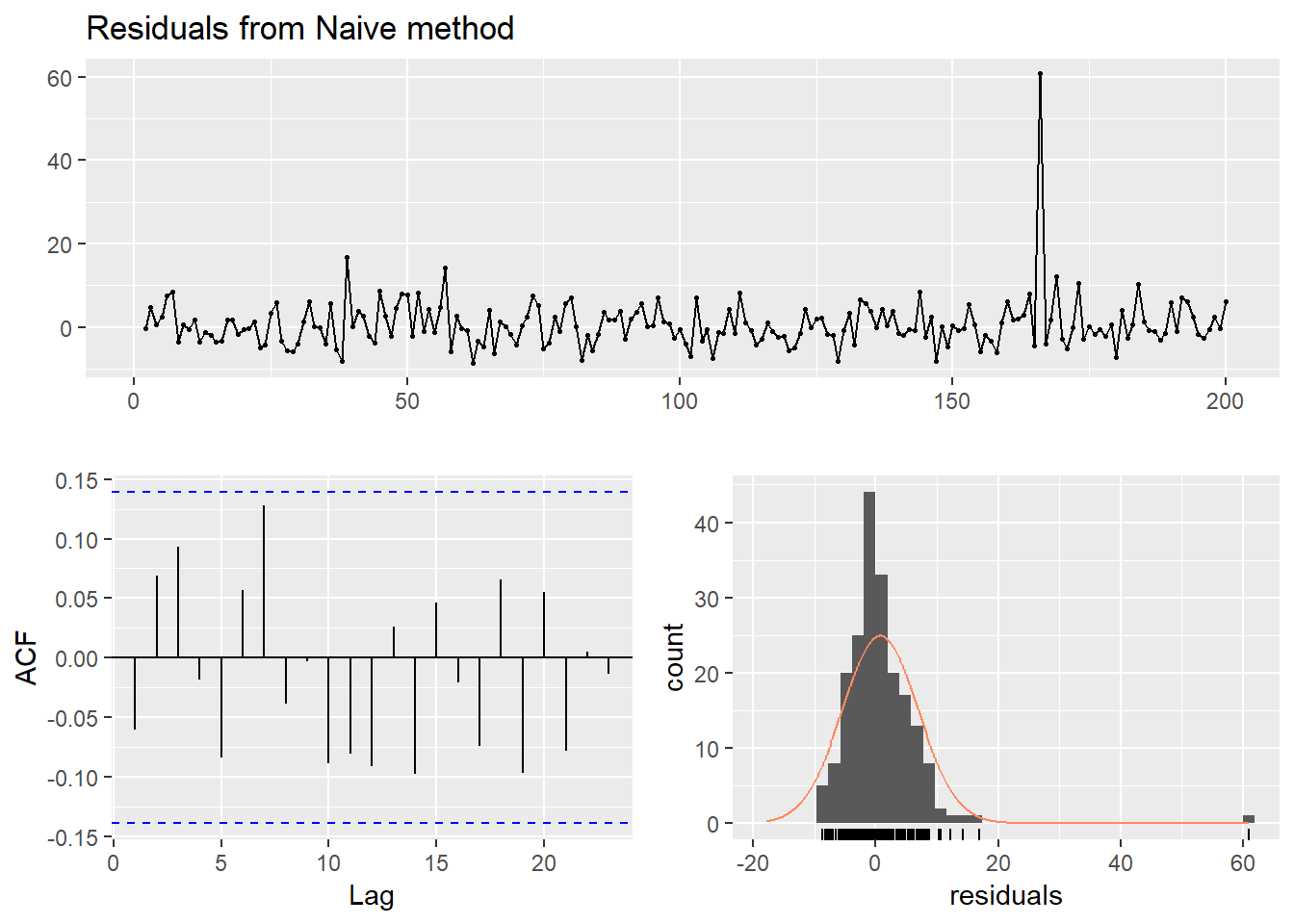## ## Ljung-Box test ## ## data: Residuals from Naive method ## Q* = 11.031, df = 10, p-value = 0.3551 ## ## Model df: 0. Total lags used: 10 ## Evaluating Forecast Accuaracy Training & Test Sets apart from windows you can use subset which you can use creatively subset1 <- subset(ausbeer, start=length(ausbeer)-4*5) # extracts last 5 years of observation subset2 <- subset(ausbeer, quarter=1) You can use head() or tail() for extracting first few or last few observations. tail(ausbeer,4*5) ## Qtr1 Qtr2 Qtr3 Qtr4 ## 2005 408 482 ## 2006 438 386 405 491 ## 2007 427 383 394 473 ## 2008 420 390 410 488 ## 2009 415 398 419 488 ## 2010 414 374 forecast errors are di|erent from residuals in two ways. First, residuals are calculated on the training set while forecast errors are calculated on the test set. Scale Dependent Errors: Mean Absolute Error ( MAE) and Root Mean Squared Error ( RMSE) Percentage Error: MAPE: Mean Absolute percentage error. Scaled Error: A scaled error is less than 1 if it arises from a forecast better than the average naive forecast. eg. beer2 <- window(ausbeer,start=1992,end=c(2007,4)) beerfit1 <- meanf(beer2,h=10) beerfit2 <- rwf(beer2,h=10) beerfit3 <- snaive(beer2,h=10) autoplot(window(ausbeer, start=1992)) + autolayer(beerfit1, series="Mean", PI=FALSE) + autolayer(beerfit2, series="Naïve", PI=FALSE) + autolayer(beerfit3, series="Seasonal naïve", PI=FALSE) + xlab("Year") + ylab("Megalitres") + ggtitle("Forecasts for quarterly beer production") + guides(colour=guide_legend(title="Forecast"))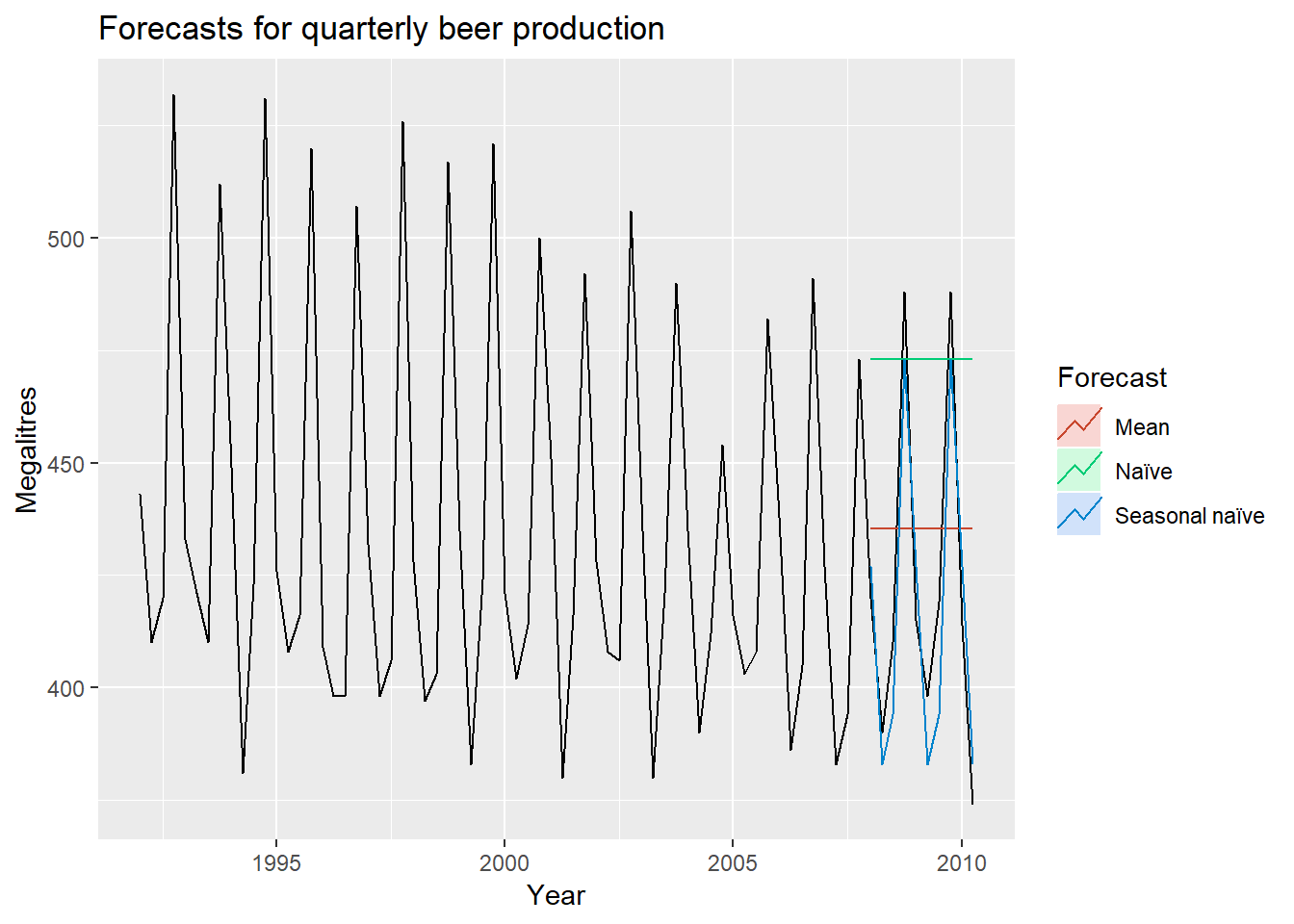Lets compute the forecast accuracy beer3 <- window(ausbeer, start=2008) accuracy(beerfit1, beer3) ## ME RMSE MAE MPE MAPE MASE ## Training set 0.000 43.62858 35.23438 -0.9365102 7.886776 2.463942 ## Test set -13.775 38.44724 34.82500 -3.9698659 8.283390 2.435315 ## ACF1 Theil's U ## Training set -0.10915105 NA ## Test set -0.06905715 0.801254 accuracy(beerfit2, beer3) ## ME RMSE MAE MPE MAPE MASE ## Training set 0.4761905 65.31511 54.73016 -0.9162496 12.16415 3.827284 ## Test set -51.4000000 62.69290 57.40000 -12.9549160 14.18442 4.013986 ## ACF1 Theil's U ## Training set -0.24098292 NA ## Test set -0.06905715 1.254009 accuracy(beerfit3, beer3) ## ME RMSE MAE MPE MAPE MASE ## Training set -2.133333 16.78193 14.3 -0.5537713 3.313685 1.0000000 ## Test set 5.200000 14.31084 13.4 1.1475536 3.168503 0.9370629 ## ACF1 Theil's U ## Training set -0.2876333 NA ## Test set 0.1318407 0.298728 It is clear that the seasonal naive is the best for this data. Lets see the Google Stock price googfc1 <- meanf(goog200, h=40) googfc2 <- rwf(goog200, h=40) googfc3 <- rwf(goog200, drift=TRUE, h=40) autoplot(subset(goog, end = 240)) + autolayer(googfc1, PI=FALSE, series="Mean") + autolayer(googfc2, PI=FALSE, series="Naïve") + autolayer(googfc3, PI=FALSE, series="Drift") + xlab("Day") + ylab("Closing Price (US$)") +
ggtitle("Google stock price (daily ending 6 Dec 13)") +
guides(colour=guide_legend(title="Forecast"))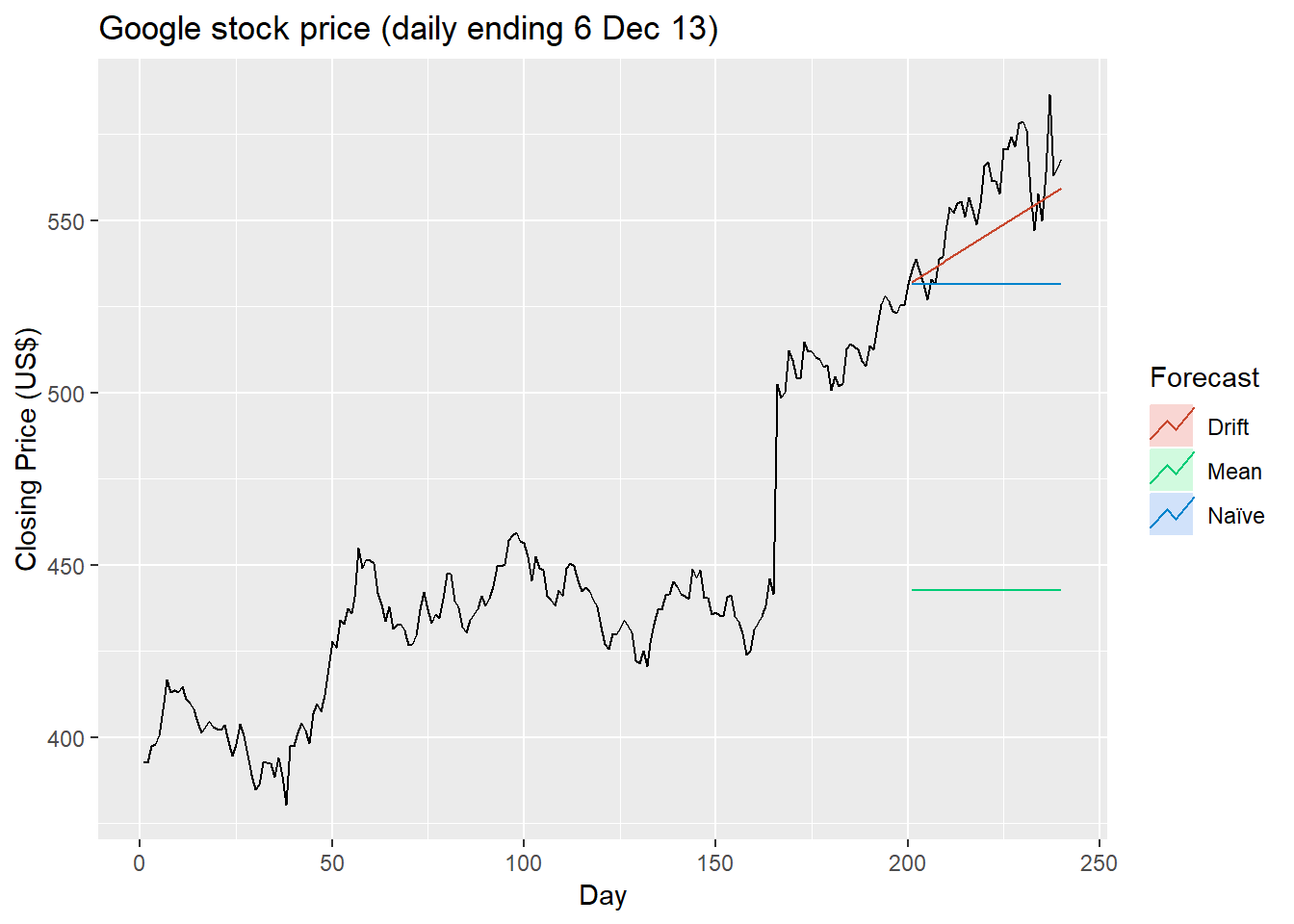Lets check the accuracy

googtest <- window(goog, start=201, end=240)
accuracy(googfc1, googtest)
##                         ME      RMSE       MAE        MPE     MAPE
## Training set -4.296286e-15  36.91961  26.86941 -0.6596884  5.95376
## Test set      1.132697e+02 114.21375 113.26971 20.3222979 20.32230
##                   MASE      ACF1 Theil's U
## Training set  7.182995 0.9668981        NA
## Test set     30.280376 0.8104340  13.92142
accuracy(googfc2, googtest)
##                      ME      RMSE       MAE       MPE      MAPE     MASE
## Training set  0.6967249  6.208148  3.740697 0.1426616 0.8437137 1.000000
## Test set     24.3677328 28.434837 24.593517 4.3171356 4.3599811 6.574582
##                     ACF1 Theil's U
## Training set -0.06038617        NA
## Test set      0.81043397  3.451903
accuracy(googfc3, googtest)
##                         ME      RMSE       MAE         MPE      MAPE
## Training set -5.998536e-15  6.168928  3.824406 -0.01570676 0.8630093
## Test set      1.008487e+01 14.077291 11.667241  1.77566103 2.0700918
##                  MASE        ACF1 Theil's U
## Training set 1.022378 -0.06038617        NA
## Test set     3.119002  0.64732736  1.709275

TIME SERIES CROSS VALIDATION

These are implemented by tsCV()

e <- tsCV(goog200, rwf, drift=TRUE, h=1)
sqrt(mean(e^2, na.rm=TRUE))
##  6.233245

The RMSE is 6.23 which is more than the accuracy naive accuracy with drift.

The code below evaluates the forecasting performance of 1- to 8-step-ahead naïve forecasts with tsCV() , using MSE as the forecast error measure.

e <- tsCV(goog200, forecastfunction=naive, h=8)
# Compute the MSE values and remove missing values
mse <- colMeans(e^2, na.rm = T)
# Plot the MSE values against the forecast horizon
data.frame(h = 1:8, MSE = mse) %>%
ggplot(aes(x = h, y = MSE)) + geom_point()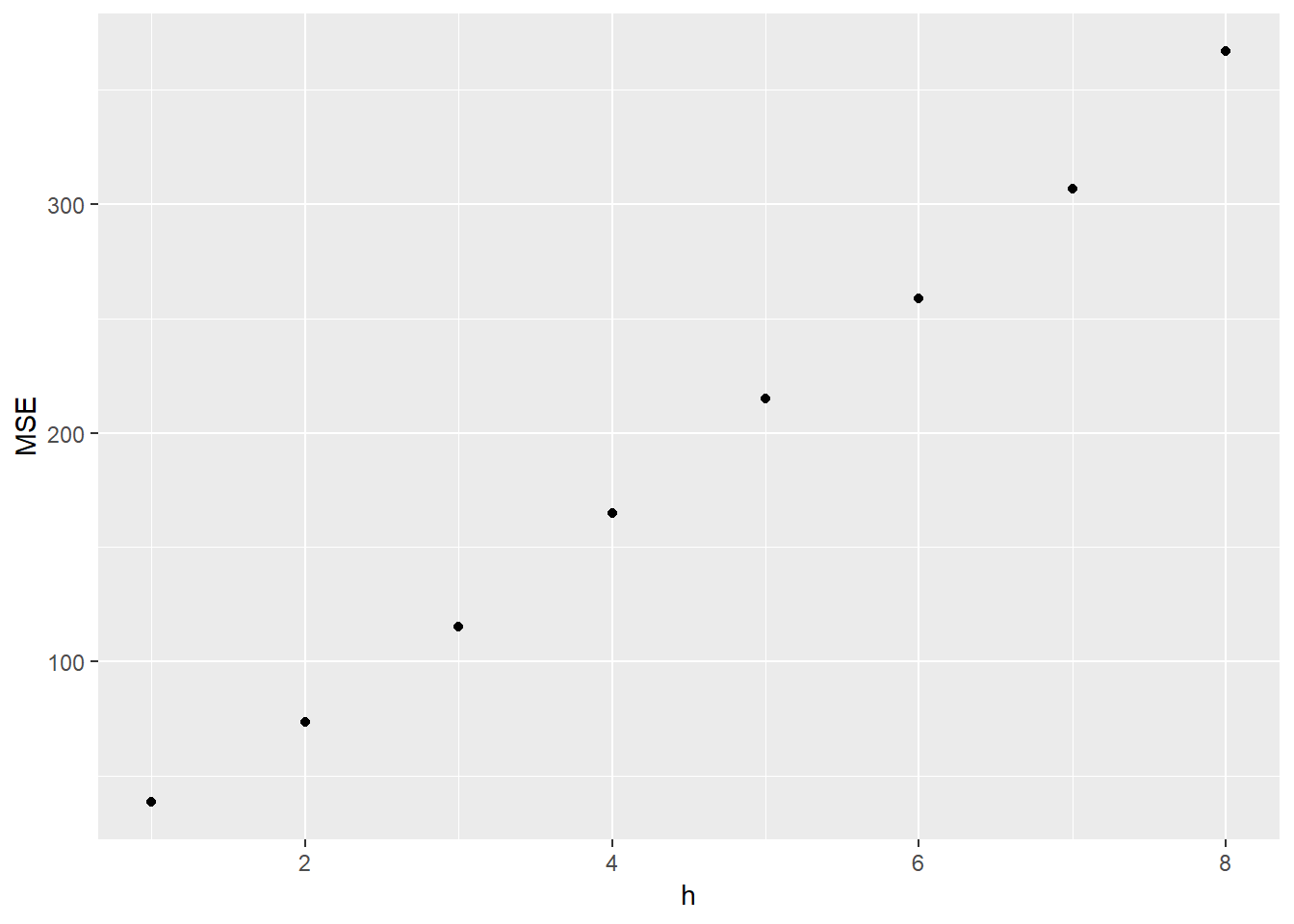The plot shows that the forecast error increases as the forecast horizon increases, as we would expect

## Prediction Intervals

They will be computed when using any of the benchmark forecasting method.

naive(goog200)
##     Point Forecast    Lo 80    Hi 80    Lo 95    Hi 95
## 201       531.4783 523.5222 539.4343 519.3105 543.6460
## 202       531.4783 520.2267 542.7298 514.2705 548.6861
## 203       531.4783 517.6980 545.2586 510.4031 552.5534
## 204       531.4783 515.5661 547.3904 507.1428 555.8138
## 205       531.4783 513.6880 549.2686 504.2704 558.6862
## 206       531.4783 511.9900 550.9666 501.6735 561.2830
## 207       531.4783 510.4285 552.5280 499.2854 563.6711
## 208       531.4783 508.9751 553.9814 497.0627 565.8939
## 209       531.4783 507.6101 555.3465 494.9750 567.9815
## 210       531.4783 506.3190 556.6375 493.0005 569.9561

When plotted these will be shaded

autoplot(naive(goog200))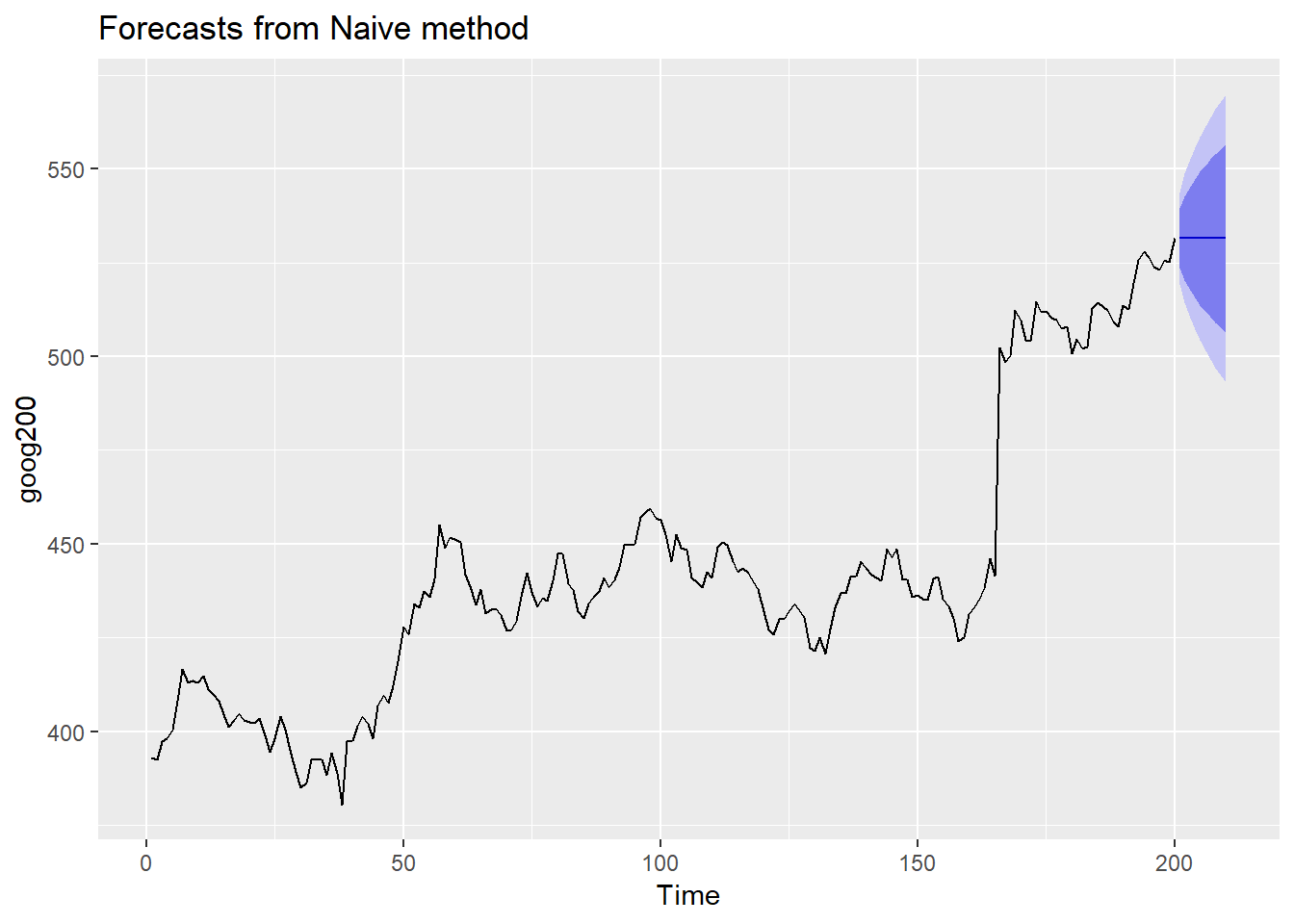You can obtain Prediction interval from bootstrap residuals.

naive(goog200, bootstrap=TRUE)
##     Point Forecast    Lo 80    Hi 80    Lo 95    Hi 95
## 201       531.4783 525.7393 537.0061 523.2307 541.3329
## 202       531.4783 523.1884 539.6327 519.4988 546.2181
## 203       531.4783 520.8690 541.4541 516.6991 551.3237
## 204       531.4783 519.1286 543.0425 514.2819 555.6406
## 205       531.4783 517.3936 544.8556 511.4128 565.8387
## 206       531.4783 515.9969 546.6482 509.0954 581.0981
## 207       531.4783 514.4178 547.8430 507.1795 583.6681
## 208       531.4783 513.2266 549.5682 505.2108 585.1323
## 209       531.4783 511.8745 550.5925 503.4841 586.6394
## 210       531.4783 510.5238 552.1635 502.2158 587.8474

In this case it is similar to the previous one.

When a transformation is used, the prediction interval should be on the transformed scale, and the end points back transformed to give a prediction interval on the original scale. this is done automatically by forecast package in R (provided you have used the lambad argument, when computing the forecast.

The forecast() function always returns objects of the class forecast.

You can apply forecast()simply

forecast(ausbeer,h=4)
##         Point Forecast    Lo 80    Hi 80    Lo 95    Hi 95
## 2010 Q3       404.6025 385.8695 423.3355 375.9529 433.2521
## 2010 Q4       480.3982 457.5283 503.2682 445.4216 515.3748
## 2011 Q1       417.0367 396.5112 437.5622 385.6456 448.4277
## 2011 Q2       383.0996 363.5063 402.6930 353.1341 413.0651

But you should not do it blindly. You will fit a model, appropriate to the data and then use forecast() to produce forecast.

save.image("workspace.Rdata")